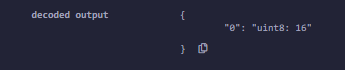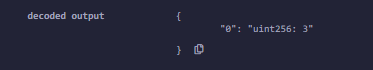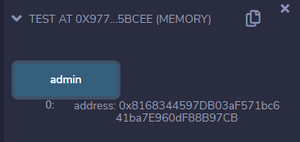GFG App
Open AppBrowser
Continue

A Variable is basically a placeholder for the data which can be manipulated at runtime. Variables allow users to retrieve and change the stored information.

### Rules For Naming Variables

1. A variable name should not match with reserved keywords.

2. Variable names must start with a letter or an underscore (_), and may contain letters from “a to z” or “A to Z” or digits from “0 to 9” and characters also.

Example:

```Geeks123, geeks, _123geeks are valid variable names
123geeks, \$Geeks, 12_geeks are invalid variable names
```

3. The name of variables are case sensitive i.e.

Example:

```Geeks123 and geeks123 are different variables
```

### Declaration of Variables

In Solidity declaration of variables is a little bit different, to declare a variable the user has to specify the data type first followed by access modifier.

Syntax:

```<type> <access modifier> <variable name> ;
```

Example:

```int public int_var;
```

### Types of Variables

Solidity is a statically typed language i.e. each declared variable always has a default value based on its data type, which means there is no concept of ‘null’ or ‘undefined’. Solidity supports three types of variables:

1. State Variables: Values of these variables are permanently stored in the contract storage. Each function has its own scope, and state variables should always be defined outside of that scope.

Example: In the below example. the contract Solidity_var_Test initializes the values of an unsigned integer state variable using a constructor.

## Solidity

 `// Solidity program to  ` `// demonstrate state  ` `// variables ` `pragma solidity ^0.5.0; ` ` `  `// Creating a contract ` `contract` `Solidity_var_Test { ` `    `  `   ``// Declaring a state variable ` `   ``uint8 ``public` `state_var;       ` ` `  `   ``// Defining a constructor ` `   ``constructor() ``public` `{ ` `      ``state_var = 16;    ` `   ``} ` `}`

Output :2. Local Variable: Values of these variables are present till the function executes and it cannot be accessed outside that function. This type of variable is usually used to store temporary values.

Example: In the below example, the contract Solidity_var_Test defines a function to declare and initialize the local variables and return the sum of the two local variables.

## Solidity

 `// Solidity program to demonstrate ` `// local variables ` `pragma solidity ^0.5.0; ` ` `  `// Creating a contract ` `contract` `Solidity_var_Test { ` ` `  `   ``// Defining function to show the declaration and ` `   ``// scope of local variables ` `   ``function getResult() ``public` `view returns(uint){ ` `      `  `      ``// Initializing local variables ` `      ``uint local_var1 = 1;  ` `      ``uint local_var2 = 2; ` `      ``uint result = local_var1 + local_var2; ` `      `  `      ``// Access the local variable ` `      ``return` `result;  ` `   ``} ` `} `

Output :3. Global Variables: These are some special variables that can be used globally and give information about the transactions and blockChain properties. Some of the global variables are listed below :

Example: In the below example, the contact Test uses the msg.sender variable to access the address of the person deploying the contract.

## Solidity

 `// Solidity program to  ` `// show Global variables ` `pragma solidity ^0.5.0; ` ` `  `// Creating a contract ` `contract` `Test {  ` `   `  `  ``// Defining a variable ` `  ``address ``public` `admin; ` `   `  `  ``// Creating a constructor to ` `  ``// use Global variable ` `  ``constructor() ``public` `{     ` `    ``admin = msg.sender;   ` `  ``} ` ` ``}`

Output:My Personal Notes arrow_drop_up